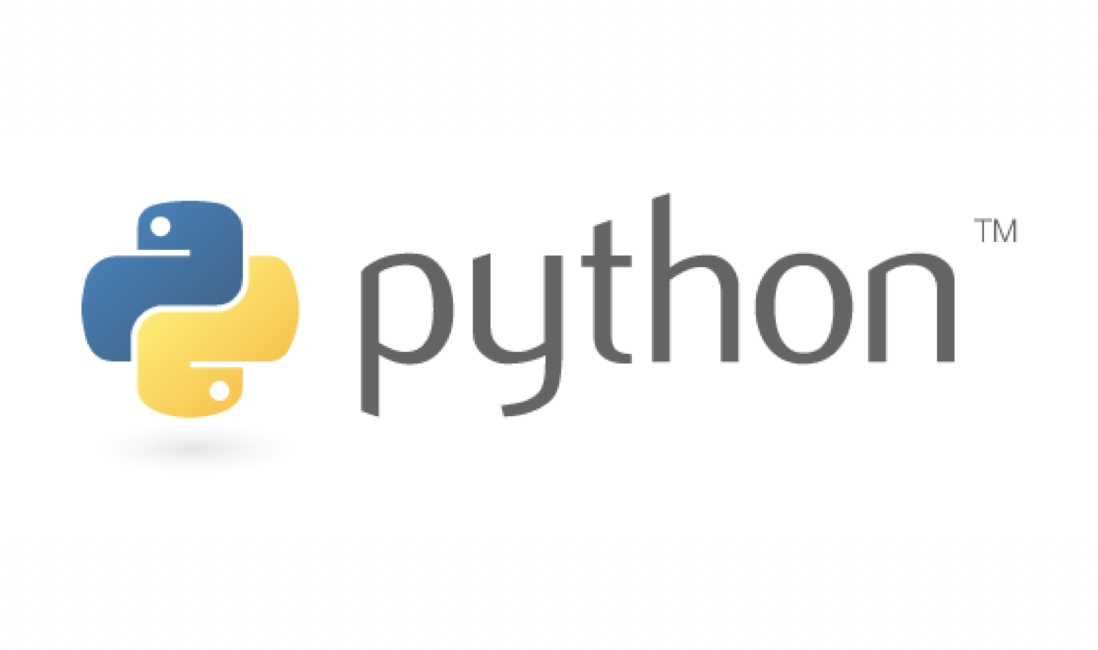### Python小技巧 - 使用不同的方式同时测试多个标记# Python小技巧 - 使用不同的方式同时测试多个标记

``````x, y, z = 1, 0, 1
if x == 1 or y == 1 or z == 1:
print('passed')``````

``````if 1 in (x, y, z):
print('passed')``````

``````if a or b or c:
print('passed')``````

``````if any((a, b, c)):
print('passed')``````